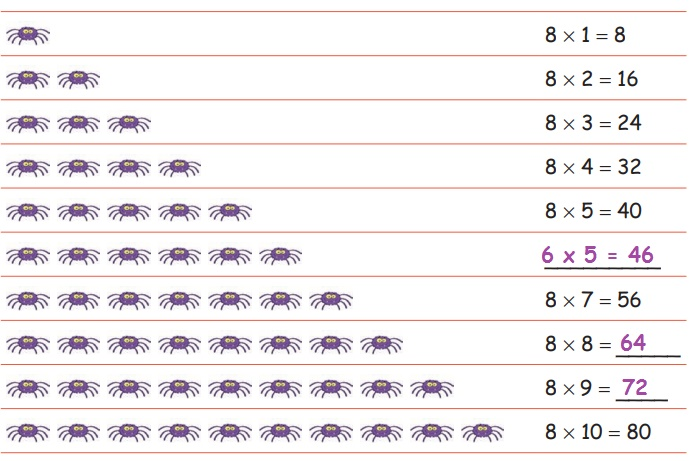Home | | Maths 4th Std | Exercise 2.4: Multiplication 8th Table

# Exercise 2.4: Multiplication 8th Table

Text Book Back Exercises Questions with Answers, Solution : 4th Maths : Term 2 Unit 2 : Numbers : Exercise 2.4: Multiplication 8th Table

Exercise 2.4: Multiplication 8th Table

Multiplication 8th Table

Complete the 8th table: Number of legs in spiderExercise 2.4

1. 8 × 4 = 32

2. 8 × 6 = 48

3. 8 × 10 = 80

4. One packet contains 8 pencils, how many pencils are there in 9 such packets?

No. of pencil in 1 packet = 8

No. of pencils in 9 packet = 9 x 8

= 72

Answer: There are 72 pencils in 9 packets.

5. The price of one ball is ₹ 10. Find the cost of 8 such balls?

Cost of 1 ball = 10

Cost of 8 balls = 10 x 8

= 80

Answer: Cost of 8 balls =  80

Tags : Numbers | Term 2 Chapter 2 | 4th Maths , 4th Maths : Term 2 Unit 2 : Numbers
Study Material, Lecturing Notes, Assignment, Reference, Wiki description explanation, brief detail
4th Maths : Term 2 Unit 2 : Numbers : Exercise 2.4: Multiplication 8th Table | Numbers | Term 2 Chapter 2 | 4th Maths